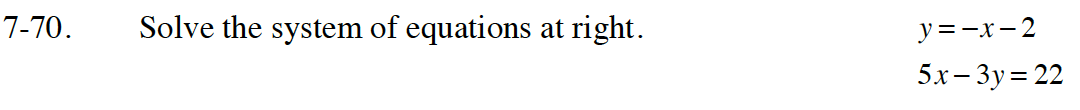Home > CC4 > Chapter 7 > Lesson 7.1.6 > Problem7-70

7-70.
1. Solve the system of equations below. Homework Help ✎

y = −x − 2
5x − 3y = 22Try using the Substitution Method because the first equation is already in y = mx + b form (slope-intercept form).